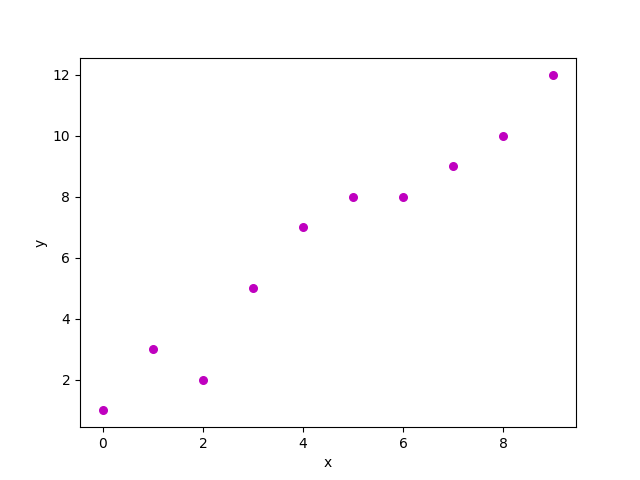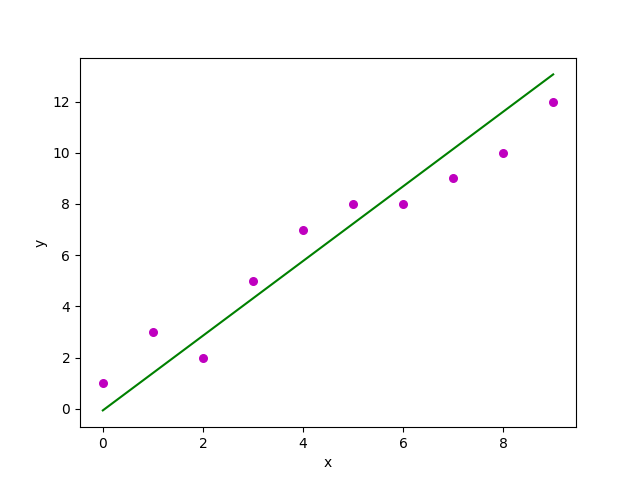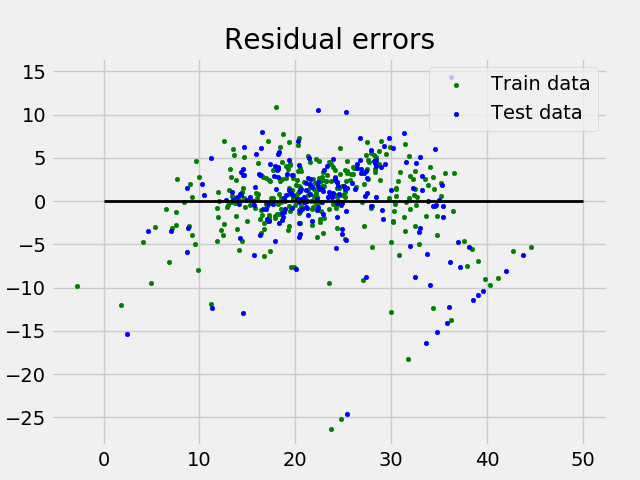# Linear Regression (Python Implementation)

This article discusses the basics of linear regression and its implementation in the Python programming language. Linear regression is a statistical method for modeling relationships between a dependent variable with a given set of independent variables.

Note: In this article, we refer to dependent variables as responses and independent variables as features for simplicity. In order to provide a basic understanding of linear regression, we start with the most basic version of linear regression, i.e. Simple linear regression.

## Simple Linear Regression

Simple linear regression is an approach for predicting a response using a single feature. It is one of the most basic machine learning models that a machine learning enthusiast gets to know about. In linear regression, we assume that the two variables i.e. dependent and independent variables are linearly related. Hence, we try to find a linear function that predicts the response value(y) as accurately as possible as a function of the feature or independent variable(x). Let us consider a dataset where we have a value of response y for every feature x:For generality, we define:

x as feature vector, i.e x = [x_1, x_2, …., x_n],

y as response vector, i.e y = [y_1, y_2, …., y_n]

for n observations (in the above example, n=10). A scatter plot of the above dataset looks like this:-Scatter plot for the randomly generated data

Now, the task is to find a line that fits best in the above scatter plot so that we can predict the response for any new feature values. (i.e a value of x not present in a dataset) This line is called a regression line. The equation of the regression line is represented as:Here,

• h(x_i) represents the predicted response value for ith observation.
• b_0 and b_1 are regression coefficients and represent the y-intercept and slope of the regression line respectively.

To create our model, we must “learn” or estimate the values of regression coefficients b_0 and b_1. And once we’ve estimated these coefficients, we can use the model to predict responses!
In this article, we are going to use the principle of Least Squares.

Now consider:Here, e_i is a residual error in ith observation. So, our aim is to minimize the total residual error. We define the squared error or cost function, J as:And our task is to find the value of b0 and b1 for which J(b0, b1) is minimum! Without going into the mathematical details, we present the result here:Where SSxy is the sum of cross-deviations of y and x:And SSxx is the sum of squared deviations of x:## Python Implementation of Linear Regression

We can use the Python language to learn the coefficient of linear regression models. For plotting the input data and best-fitted line we will use the matplotlib library. It is one of the most used Python libraries for plotting graphs.

## Python3

 import numpy as np import matplotlib.pyplot as plt   def estimate_coef(x, y):     # number of observations/points     n = np.size(x)       # mean of x and y vector     m_x = np.mean(x)     m_y = np.mean(y)       # calculating cross-deviation and deviation about x     SS_xy = np.sum(y*x) - n*m_y*m_x     SS_xx = np.sum(x*x) - n*m_x*m_x       # calculating regression coefficients     b_1 = SS_xy / SS_xx     b_0 = m_y - b_1*m_x       return (b_0, b_1)   def plot_regression_line(x, y, b):     # plotting the actual points as scatter plot     plt.scatter(x, y, color = "m",                marker = "o", s = 30)       # predicted response vector     y_pred = b + b*x       # plotting the regression line     plt.plot(x, y_pred, color = "g")       # putting labels     plt.xlabel('x')     plt.ylabel('y')       # function to show plot     plt.show()   def main():     # observations / data     x = np.array([0, 1, 2, 3, 4, 5, 6, 7, 8, 9])     y = np.array([1, 3, 2, 5, 7, 8, 8, 9, 10, 12])       # estimating coefficients     b = estimate_coef(x, y)     print("Estimated coefficients:\nb_0 = {}  \           \nb_1 = {}".format(b, b))       # plotting regression line     plot_regression_line(x, y, b)   if __name__ == "__main__":     main()

Output:

Estimated coefficients:
b_0 = -0.0586206896552
b_1 = 1.45747126437

And the graph obtained looks like this:Scatterplot of the points along with the regression line

## Multiple linear regression

Multiple linear regression attempts to model the relationship between two or more features and a response by fitting a linear equation to the observed data.
Clearly, it is nothing but an extension of simple linear regression. Consider a dataset with p features(or independent variables) and one response(or dependent variable).
Also, the dataset contains n rows/observations.

We define:

X (feature matrix) = a matrix of size n X p where xij denotes the values of the jth feature for ith observation.

So,and

y (response vector) = a vector of size n where y_{i} denotes the value of response for ith observation.The regression line for p features is represented as:where h(x_i) is predicted response value for ith observation and b_0, b_1, …, b_p are the regression coefficients. Also, we can write:where e_i represents a residual error in ith observation. We can generalize our linear model a little bit more by representing feature matrix X as:So now, the linear model can be expressed in terms of matrices as:where,andNow, we determine an estimate of b, i.e. b’ using the Least Squares method. As already explained, the Least Squares method tends to determine b’ for which total residual error is minimized.
We present the result directly here:where ‘ represents the transpose of the matrix while -1 represents the matrix inverse. Knowing the least square estimates, b’, the multiple linear regression model can now be estimated as:where y’ is the estimated response vector.

## Python implementation of multiple linear regression techniques

For multiple linear regression, we will use the Boston house pricing dataset.

## Python3

 from sklearn.model_selection import train_test_split import matplotlib.pyplot as plt import numpy as np from sklearn import datasets, linear_model, metrics   # load the boston dataset data_url = "http://lib.stat.cmu.edu/datasets/boston" raw_df = pd.read_csv(data_url, sep="\s+",                      skiprows=22, header=None) X = np.hstack([raw_df.values[::2, :],                raw_df.values[1::2, :2]]) y = raw_df.values[1::2, 2]   # splitting X and y into training and testing sets X_train, X_test,\     y_train, y_test = train_test_split(X, y,                                        test_size=0.4,                                        random_state=1)   # create linear regression object reg = linear_model.LinearRegression()   # train the model using the training sets reg.fit(X_train, y_train)   # regression coefficients print('Coefficients: ', reg.coef_)   # variance score: 1 means perfect prediction print('Variance score: {}'.format(reg.score(X_test, y_test)))   # plot for residual error   # setting plot style plt.style.use('fivethirtyeight')   # plotting residual errors in training data plt.scatter(reg.predict(X_train),             reg.predict(X_train) - y_train,             color="green", s=10,             label='Train data')   # plotting residual errors in test data plt.scatter(reg.predict(X_test),             reg.predict(X_test) - y_test,             color="blue", s=10,             label='Test data')   # plotting line for zero residual error plt.hlines(y=0, xmin=0, xmax=50, linewidth=2)   # plotting legend plt.legend(loc='upper right')   # plot title plt.title("Residual errors")   # method call for showing the plot plt.show()

Output:

Coefficients:
[ -8.80740828e-02   6.72507352e-02   5.10280463e-02   2.18879172e+00
-1.72283734e+01   3.62985243e+00   2.13933641e-03  -1.36531300e+00
2.88788067e-01  -1.22618657e-02  -8.36014969e-01   9.53058061e-03
-5.05036163e-01]
Variance score: 0.720898784611

and Residual Error plot looks like this:Residual Error Plot for the Multiple Linear Regression

In the above example, we determine the accuracy score using Explained Variance Score. We define:

explained_variance_score = 1 – Var{y – y’}/Var{y}

where y’ is the estimated target output, y is the corresponding (correct) target output, and Var is Variance, the square of the standard deviation. The best possible score is 1.0, lower values are worse.

## Assumptions We Make in a Linear Regression Model:

Given below are the basic assumptions that a linear regression model makes regarding a dataset on which it is applied:

• Linear relationship: The relationship between response and feature variables should be linear. The linearity assumption can be tested using scatter plots. As shown below, 1st figure represents linearly related variables whereas variables in the 2nd and 3rd figures are most likely non-linear. So, 1st figure will give better predictions using linear regression.Linear relationship i the feature space

• Little or no multi-collinearity: It is assumed that there is little or no multicollinearity in the data. Multicollinearity occurs when the features (or independent variables) are not independent of each other.
• Little or no autocorrelation: Another assumption is that there is little or no autocorrelation in the data. Autocorrelation occurs when the residual errors are not independent of each other. You can refer here for more insight into this topic.
• Homoscedasticity: Homoscedasticity describes a situation in which the error term (that is, the “noise” or random disturbance in the relationship between the independent variables and the dependent variable) is the same across all values of the independent variables. As shown below, figure 1 has homoscedasticity while Figure 2 has heteroscedasticity.Homoscedasticity in Linear Regression

As we reach the end of this article, we discuss some applications of linear regression below.

## Applications of Linear Regression:

• Trend lines: A trend line represents the variation in quantitative data with the passage of time (like GDP, oil prices, etc.). These trends usually follow a linear relationship. Hence, linear regression can be applied to predict future values. However, this method suffers from a lack of scientific validity in cases where other potential changes can affect the data.
• Economics: Linear regression is the predominant empirical tool in economics. For example, it is used to predict consumer spending, fixed investment spending, inventory investment, purchases of a country’s exports, spending on imports, the demand to hold liquid assets, labor demand, and labor supply.
• Finance: The capital price asset model uses linear regression to analyze and quantify the systematic risks of an investment.
• Biology: Linear regression is used to model causal relationships between parameters in biological systems.

Whether you're preparing for your first job interview or aiming to upskill in this ever-evolving tech landscape, GeeksforGeeks Courses are your key to success. We provide top-quality content at affordable prices, all geared towards accelerating your growth in a time-bound manner. Join the millions we've already empowered, and we're here to do the same for you. Don't miss out - check it out now!

Previous
Next